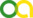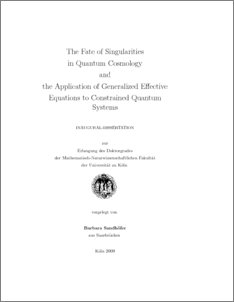Sandhöfer, Barbara (2009). The Fate of Singularities in Quantum Cosmology and the Application of Generalized Effective Equations to Constrained Quantum Systems. PhD thesis, Universität zu Köln.Preview
PDF
DissertationBSandhoefer.pdf

## Abstract

The first part of the thesis is concerned with the fate of singularities in quantum cosmology. The second part addresses the derivation of predictions from quantum cosmology. In the first part, two classes of cosmological models were studied. In the first class of models, the universe evolves to or emerges from a big-rip singularity. Here, energy density, pressure and scale factor diverge after a finite amount of time. This type of singularity arises rather generically in cosmological models with phantom dark energy. For each of these phantom-field models, the corresponding scenario with ordinary scalar field was studied. The scalar field induced a big-bang singularity. The second class of models studied was dominated by a big-brake singularity. At the big brake, the universe evolution comes to a halt due to an infinite deceleration. The motivation behind this choice of models was the occurrence of a singularity at large scale factor. The major question pursued was whether these types of singularity were resolved on the quantum level. If such singularities were resolved in quantum cosmology, this would imply that quantum gravitational effects can occur in the macroscopic universe. After devising classical models that contain the respective singularity, I subjected these models to quantization which was carried out in the geometrodynamical approach. The governing equation is then the Wheeler�DeWitt equation. I found solutions to the Wheeler�DeWitt equation, in one case even an exact solution. Wave packets were constructed around trajectories which, on the classical level, would lead into the singularity. I have then shown that the classical trajectory can indeed be recovered from these packets through the principle of constructive interference. As criteria for singularity avoidance the vanishing of the wave function at the location of the classical singularity, as well as the spreading of wave packets upon approach of this region was used. Whereas the former ensures that the classical singularity does not contribute to the quantum theory, the latter signals a dissolution of the semi-classical approximation and thus of spacetime. In all cases, I found singularity resolution. In the case of the big-bang and big-brake singularities, the wave function vanishes at the classical singularity. These two have in common that they occur at finite value of the configuration space variables. A spreading of the wave packet is however only observed upon approach of the big-brake singularity. At the location of the classical big-rip singularity, a strict vanishing cannot be found. This singularity is located at the infinite boundary of configuration space. The wave packet spreads upon approach of this singularity. The second part of my thesis deals with the application of the generalized effective-equation scheme to constrained systems. Generalized effective equations describe a quantum system via expectation values of fundamental operators and higher moments of the wave function � instead of using the wave function itself. It is thus a very useful scheme for the derivation of predictions from quantum cosmology, e.g. in the form of corrections to classical equations of motion. The theory is formulated on a, generally, infinite-dimensional quantum phase space. The first task was to find a for- mulation of Dirac�s constraint-quantization condition on this phase space. Such a formulation was found and proven to remove degrees of freedom appropriately in the case of a single linear constraint. This result ensures the correct removal of degrees of freedom for any singly constrained system at least locally. In a second step, the newly formulated constraints � there are actually infinitely many of them � had to be consistently approximated. Such an approximation is necessary to reduce the infinite number of con- straints to a finite one. Only then can information be extracted from the system. Such an approximation scheme for non-relativistic systems was developed. Its consistency was explicitly checked using the parametrised, free non-relativistic particle.

Item Type: Thesis (PhD thesis)
Translated title:
TitleLanguage
Das Schicksal von Singularitäten in der Quantenkosmologie und die Anwendung generalisierter effektiver Gleichungen auf Systeme mit ZwangsbedingungenGerman
Creators:
CreatorsEmailORCIDORCID Put Code
Sandhöfer, Barbarabs324@thp.uni-koeln.deUNSPECIFIEDUNSPECIFIED
URN: urn:nbn:de:hbz:38-29556
Date: 2009
Language: English
Faculty: Faculty of Mathematics and Natural Sciences
Divisions: Faculty of Mathematics and Natural Sciences > Department of Physics > Institute for Theoretical Physics
Subjects: Physics
Uncontrolled Keywords:
KeywordsLanguage
Quantenkosmologie , Singularitäten , Effektive Gleichungen , Singularitätsvermeidung, QuantengravitationGerman
Quantum cosmology , singularity , effective equations , singularity avoidance , quantum gravityEnglish
Date of oral exam: 20 April 2009
Referee:View Item